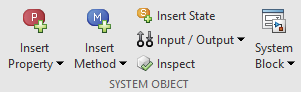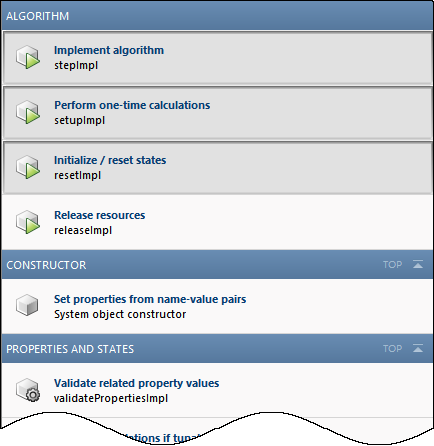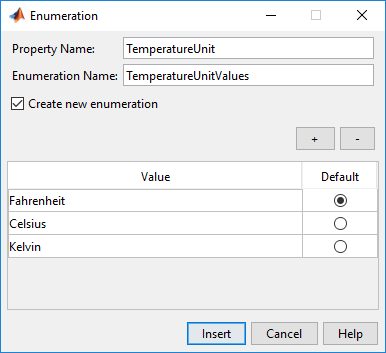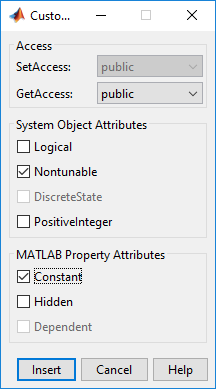## 使用 MATLAB 编辑器插入 System object 代码

### 通过代码插入定义 System object``` properties(Nontunable) Property end```

MATLAB 编辑器会插入具有默认名称 `Property` 的新属性，您可以对该名称进行重命名。如果您的现有属性组带有 `Nontunable` 特性，则 MATLAB 编辑器会将新属性插入到该组中。如果您没有属性组，MATLAB 编辑器将创建一个具有正确特性的属性组。

 属性 System object 的属性：数值、逻辑、枚举、正整数、可调数值、私有、受保护和自定义。当您选择“枚举”或“自定义”属性时，将打开一个单独的对话框，以指导您创建这些属性。 方法 System object 定义中的常用方法。MATLAB 编辑器只会创建方法结构体。您需要指定该方法的操作。 插入方法菜单按类别来整理方法，例如算法、输入和输出以及属性和状态。当您从该菜单中选择一种方法时，MATLAB 编辑器会将方法模板插入到 System object 代码中。在此示例中，选择插入方法 > 释放资源将插入以下代码：``` function releaseImpl(obj) % Release resources, such as file handles end```如果插入方法菜单中的某个方法已存在于 System object 代码中，则该方法在插入方法菜单中灰显：状态 包含 `DiscreteState` 特性的属性。 输入/输出 输入、输出以及相关方法，例如验证输入和不允许更改输入大小。当您选择某个输入或输出时，MATLAB 编辑器将在 `stepImpl` 方法中插入指定代码。在此示例中，选择插入 > 输入后，MATLAB 编辑器将插入所需的输入变量 `u2`。MATLAB 编辑器会确定变量名称，但您可以在插入变量后对其进行更改。``` function y = stepImpl(obj,u,u2) % Implement algorithm. Calculate y as a function of % input u and discrete states. y = u; end```

### 创建温度枚举属性

1. 打开一个新的或现有的 System object。

2. 在 MATLAB 编辑器中，选择插入属性 > 枚举

3. 枚举对话框中，输入：

1. 属性名称`TemperatureUnit`

2. 枚举名称`TemperatureUnitValues`

4. 选中创建新枚举复选框。

5. 使用 （减号）按钮删除现有枚举值。

6. 使用 （加号）按钮和以下值添加三个枚举值：

• `Fahrenheit`

• `Celsius`

• `Kelvin`

7. 点击默认选择 `Fahrenheit` 作为默认值。

该对话框现在看上去如下所示：8. 要创建此枚举和关联的类，请点击

9. 在 MATLAB 编辑器中，将创建具有该枚举定义的附加类文件。将该枚举类定义文件另存为 `TemperatureUnitValues.m`

```classdef TemperatureUnitValues < int32 enumeration Fahrenheit (0) Celsius (1) Kelvin (2) end end```

在 System object 类定义中，添加了以下代码：

``` properties(Nontunable) TemperatureUnit (1, 1) TemperatureUnitValues = TemperatureUnitValues.Fahrenheit end```

### 为冻结点创建自定义属性

1. 打开一个新的或现有的 System object。

2. 在 MATLAB 编辑器中，选择插入属性 > 自定义属性

3. 在“自定义属性”对话框的 System object 属性下，选择 Nontunable。在 MATLAB 属性特性下，选择 Constant。将 GetAccess 保留设置为 “`public`”。SetAccess 将被灰显，因为无法使用 System object 方法来设置类型为常量的属性。4. 点击，并将以下代码插入到 System object 定义中：

``` properties(Nontunable, Constant) Property end```
5. `Property` 替换为您的属性。

``` properties(Nontunable, Constant) FreezingPointFahrenheit = 32; end```

### 添加方法来验证输入

1. 打开一个新的或现有的 System object。

2. 在 MATLAB 编辑器中，选择插入方法 > 验证输入

MATLAB 编辑器会将以下代码插入到 System object 中：

``` function validateInputsImpl(obj,u) % Validate inputs to the step method at initialization end```